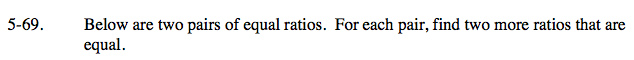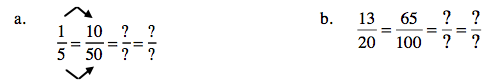### Home > CAAC > Chapter 5 > Lesson 5.2.1 > Problem5-69

5-69.To get an equal ratio, multiply the first ratio by 1.

$\frac{3}{3}=1$

$\left(\frac{1}{5}\right)\left(\frac{3}{3}\right)=\frac{3}{15}$